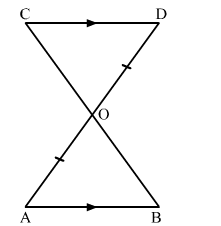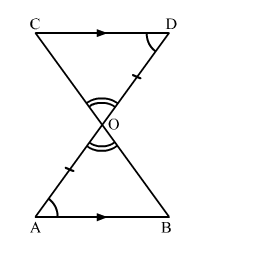# In the given figure, AB || CD and O is the midpoint of AD.

Question:

In the given figure, AB || CD and O is the midpoint of AD.
Show that
(i) ΔAOB ≅ ΔDOC.
(ii) O is the midpoint of BCSolution:Given: In the given figure, AB || CD and O is the midpoint of AD.

To prove:
(i) ΔAOB ≅ ΔDOC.
(ii) O is the midpoint of BC.

Proof:
(i) In ΔAOB and ΔDOC,
BAO = ∠CDO                    (Alternate interior angles, AB || CD)
AO = DO                              (Given, O is the midpoint of AD)
AOB = ∠DOC                   (Vertically opposite angles)

∴ By ASA congruence criteria,
ΔAOB ≅ ΔDOC

(ii) ∵ ΔAOB ≅ ΔDOC           [From (i)]
∴ BO = CO                           (CPCT)
Hence, O is the midpoint of BC.